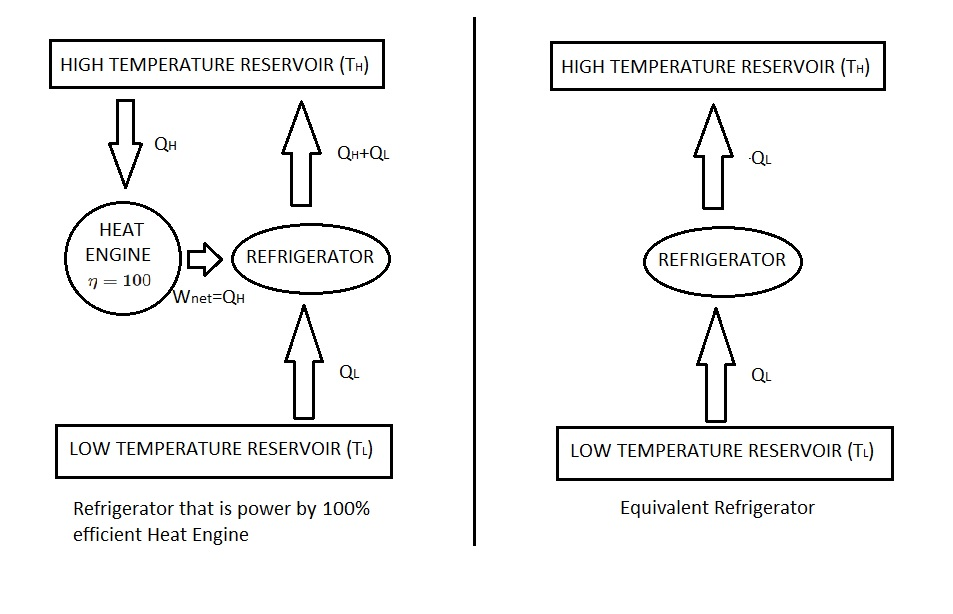# Clausius Statement vs Kelvin-Planck Statement

The 2nd law of thermodynamics can be described by two classical statements.  One of these statements is called the Kelvin-Planck statement.  This statements is related to heat engines.  In addition to the Kelvin-Planck statement, the the Clausius statement also describes the 2nd law.  This statement is related to refrigerators and heat pumps.

### Kelvin-Planck Statement

First,  the Kelvin-Planck statement is expressed as the following.

“It is impossible for any device that operates on a cycle to receive heat from a single reservoir and produce a net amount of work.”

As a result, a heat engine must exchange heat from a high temperature source to a low temperature sink.

### Clausius Statement

Next, the Clausius Statement is expressed as the following.

“It is impossible to construct a device that operates in a cycle and produces no effect other than the transfer of heat from a lower-temperature body to a higher temperature body.”

In other words,  this statement is acknowledging that heat will not transfer from a cold medium to a warm one under its own volition.  However, it does not say that such a device is not impossible to construct.  Instead it is stating that in order for this occur work must implied.  For a refrigerator, or heat pump, work is applied using a compressor that is driven from an external power source.

### Comparing the Two Statements

Both the Kelvin-Planck Statement and Clausius Statement are negative statements.  A negative statement is a statement that cannot be proved.  However, to this date there has not been an experiment that disproves the 2nd law of thermodynamics.  As a result, this is taken as sufficient proof of validity.  Due to this fact if a device violates the Clausius Statement than it will also violate the the Kelvin-Planck Statement, or vise versa.

For example let consider a heat-engine-refrigerator combination that is shown in the following images.First, let’s take a look at the Kelvin-Planck statement.  According to this statement a heat engine cannot have 100% efficiency. Instead it must exchange a certain amount of heat from a high temperature source to a low temperature sink.   However, the image is showing that the heat engine is converting all of $Q_H$ to work which can only occur if it has 100% efficiency.  Hence, it is in violation of the Kelvin-Planck statement.

Next, let’s take a look a the Clausius statement.  In order to respect this statement work energy from an outside source must be applied.  According to the image, all of $Q_H$  is converted to work.  This is than used to run the refrigerator to remove a certain amount of heat $Q_L$ from the low temperature reservoir.  By doing this $Q_L+Q_H$ will be rejected to the high temperature reservoir.  In turn, the high temperature reservoir receives a net amount of heat $Q_L$.  This means that work is not required to run this refrigerator, violating Clausius statement.

As shown, if the Clausius statement is violated than the Kelvin-Planck statement is also violated.  This means that both statements are equivalent expressions for the second law of thermodynamics.